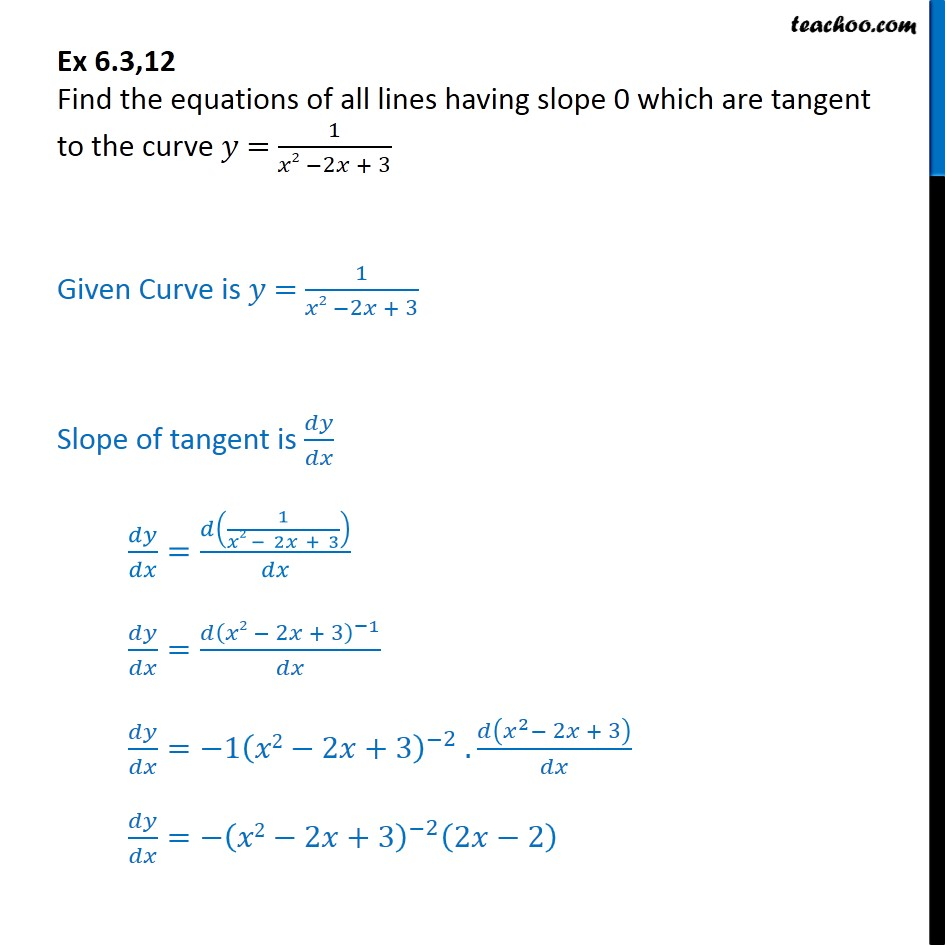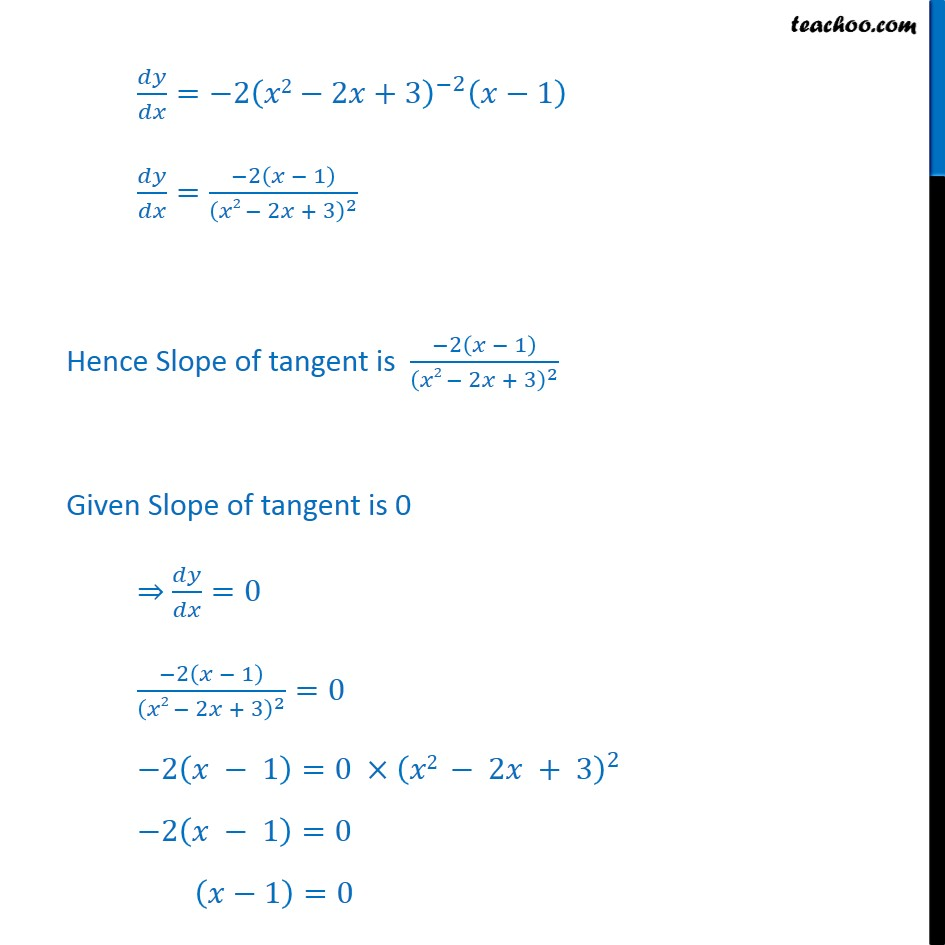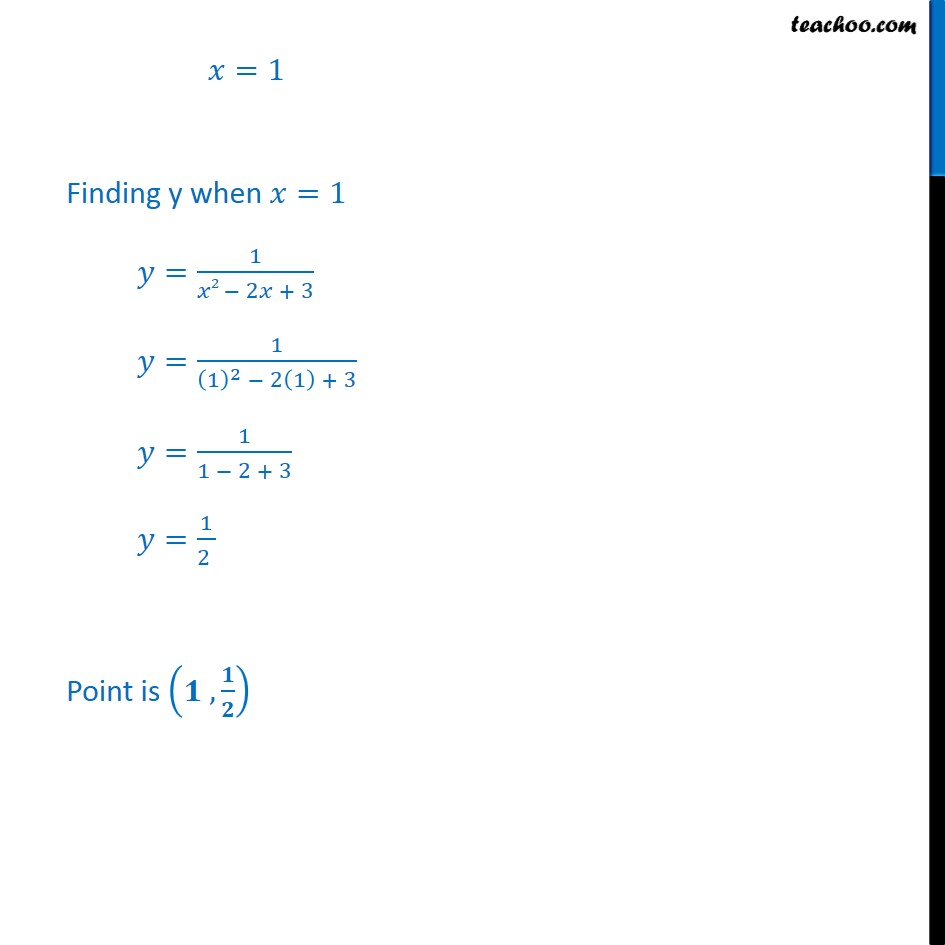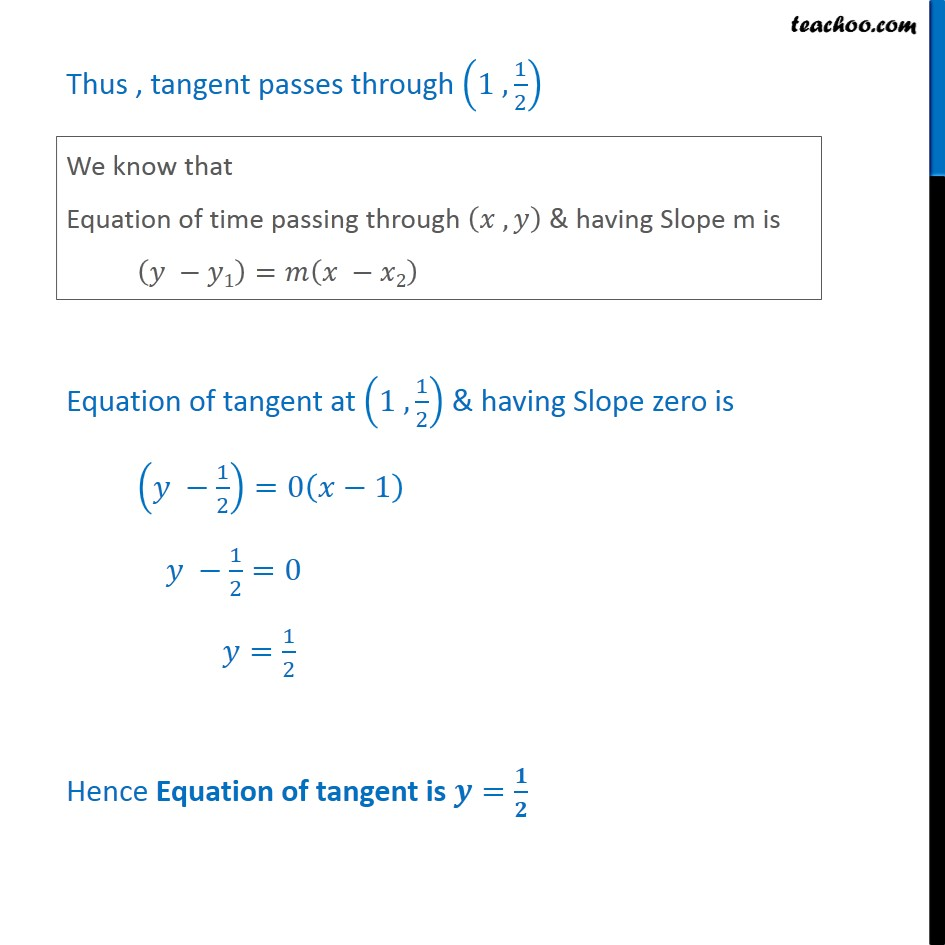1. Class 12
2. Important Question for exams Class 12
3. Chapter 6 Class 12 Application of Derivatives

Transcript

Ex 6.3,12 Find the equations of all lines having slope 0 which are tangent to the curve = 1 2 2 + 3 Given Curve is = 1 2 2 + 3 Slope of tangent is = 1 2 2 + 3 = 2 2 + 3 1 = 1 2 2 +3 2 . 2 2 + 3 = 2 2 +3 2 2 2 = 2 2 2 +3 2 1 = 2 1 2 2 + 3 2 Hence Slope of tangent is 2 1 2 2 + 3 2 Given Slope of tangent is 0 =0 2 1 2 2 + 3 2 =0 2 1 =0 2 2 + 3 2 2 1 =0 1 =0 =1 Finding y when =1 = 1 2 2 + 3 = 1 1 2 2 1 + 3 = 1 1 2 + 3 = 1 2 Point is , Thus , tangent passes through 1 , 1 2 Equation of tangent at 1 , 1 2 & having Slope zero is 1 2 =0 1 1 2 =0 = 1 2 Hence Equation of tangent is =

Chapter 6 Class 12 Application of Derivatives

Class 12
Important Question for exams Class 12